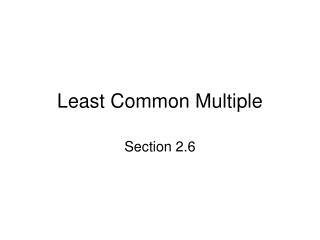DownloadDownload PresentationLeast Common Multiple

# Least Common Multiple

Download Presentation## Least Common Multiple

- - - - - - - - - - - - - - - - - - - - - - - - - - - E N D - - - - - - - - - - - - - - - - - - - - - - - - - - -
##### Presentation Transcript

1. Least Common Multiple Section 2.6

2. Objectives • Find the least common multiple (LCM) of two or more whole numbers using the Individual Prime-Factoring Method • Find the least common multiple (LCM) of two or more whole numbers using the Group Prime-Factoring Method

3. Objective 1Find the least common multiple (LCM) of two or more whole numbers using the Individual Prime-Factoring Method

4. Objective 1 • Four ways to define LCM • Smallest natural number that is a multiple of each whole number • Smallest natural number has each whole number as a factor • Smallest natural number has each whole number as a divisor • Smallest natural number that each whole number will divide evenly • Could list the multiples of each number and select the smallest that is common to all

5. Objective 1 • To write the LCM of two or more whole numbers using the Individual Price-Factoring Method • Find the prime factorization of each number in exponent form • Find the highest power of each prime factor

6. Objective 2:Find the least common multiple (LCM) of two or more whole numbers using the Group Prime-Factoring Method

7. Objective 2 • Divide at least two of the numbers by any prime number, Continue dividing the remaining quotients in the same manner until no two quotients have a common divisor • Write the product of the divisors and the remaining quotients